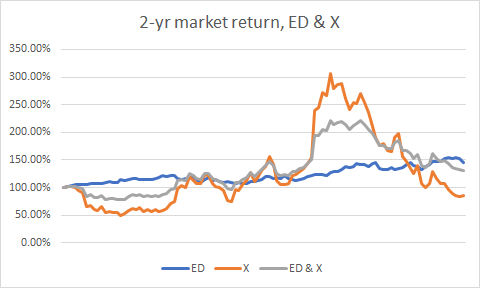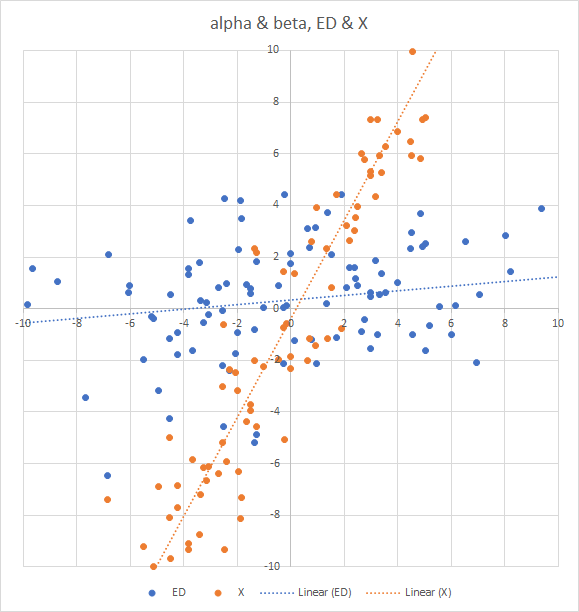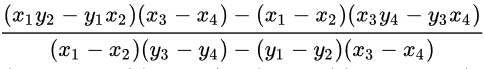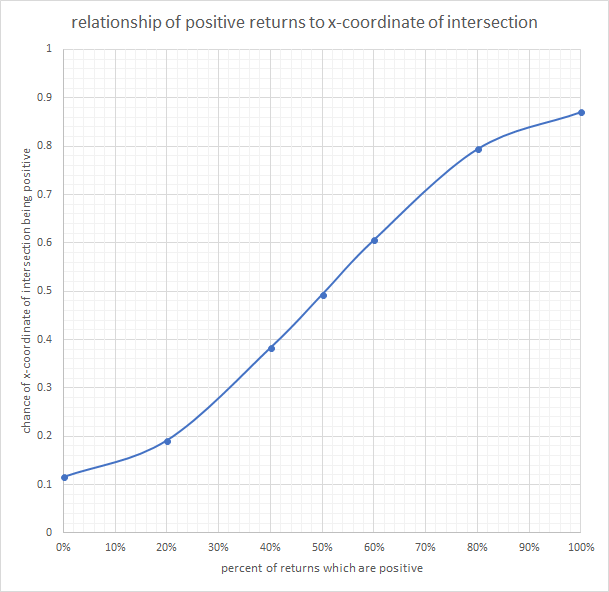# Why Low Beta Outperforms

Jun. 15, 2018 9:27 AM ET50 Comments

## Summary

• Lays out what beta is, how it has been used, and why it doesn’t correlate with returns.
• Explains why low-beta assets outperform high-beta assets without regard to investor behavior.
• Takes apart the Capital Asset Pricing Model and shows, mathematically, why it is fundamentally flawed.
• Proves that low-beta strategies will always offer higher alpha.
• Recommends investing in assets that are relatively insensitive to the market as a whole.

For almost fifty years people have written about a “low-beta anomaly,” and despite a lot of literature on the subject, nobody has been able to figure out what exactly causes it.

The Capital Asset Pricing Model (CAPM), which was developed in the 1960s by William Sharpe (the inventor of the Sharpe ratio and the winner of the 1990 Nobel prize in economics) and John Lintner, proposes that the expected return on an asset is the risk-free rate plus the product of the market return and the asset’s beta, or its systemic risk. Beta is calculated as the slope of the linear regression line formed by the asset’s return plotted against the market return. According to this model, the higher the beta, the higher the expected return should be. This is the model that is still used widely in business (for estimating the cost of capital) and among financial advisors in evaluating the performance of portfolios. It’s the centerpiece of many MBA courses, and is one of very few asset-pricing models available. (The main alternative is Arbitrage Pricing Theory, a multi-factor model.)

I’ll try to explain the CAPM in layman’s terms. Choose a stock in the S&P 500. List its monthly returns over a three-year period in one column, and the monthly returns of the S&P 500 in another. Create a scatter plot of those returns, with the stock’s returns on the y-axis and the market returns on the x-axis. Now draw a straight line through that scatter plot that is the best fit according to the least-squares method (in other words, the squares of the vertical distances between the line and all the points is minimized). The slope of that line is the stock’s beta, and the y-intercept is its alpha. If the stock’s beta is 2.0, then every time the market goes up 2%, the stock will go up approximately 4% plus its alpha, and every time the market goes down 2%, the stock will go down approximately 4% minus its alpha. If the stock’s beta is 0.5, then every time the market goes up 2%, the stock will go up approximately 1% plus its alpha, and every time the market goes down 2%, the stock will go down approximately 1% minus its alpha. The CAPM states that in an efficient market, alpha will be equivalent to the risk-free rate and that therefore the higher the stock’s beta, the higher the stock’s return will be. Since beta is a measurement of volatility - high-beta stocks move a lot more than low-beta stocks - CAPM accords with the popular belief that the riskier the investment, the higher the return. And it is meant to apply to other investable assets besides stocks.

Only a few years after its development, however, a fundamental problem with the model emerged. By 1972, Merton Miller (who would share the Nobel Prize with Sharpe) and Myron Scholes (who co-originated the Black-Scholes options pricing model) had shown empirically that high-beta assets tended to have negative alphas and that low-beta assets tended to have positive alphas (and alpha correlates extremely closely with total returns). Their findings were confirmed that same year by Fischer Black (the other co-originator of the options pricing model) and Michael Jensen (who came up with the idea of alpha), and have frequently been confirmed since. Across asset classes and in different markets, alpha and beta are negatively correlated. The “riskier” assets have lower returns than the less risky ones.

A large number of explanations of this “low-beta anomaly” have since been proposed. They include leverage constraints, asset managers’ benchmarking, lottery demand, opinion differences, correction of market-driven overpricing, and so on. All these explanations have one thing in common: they’re behavioral. They look at investor behavior as basically illogical, or flawed in some way, or inefficient. I’m not going to go into these explanations in this article, because they’re all basically wrong.

Instead, I have solved this long-standing problem in a very different way. In this article I am going to show that the solution to this anomaly is purely mathematical and has nothing to do with investor behavior.

Here’s the problem as stated in mathematical terms. If you find the next two paragraphs hard to follow, feel free to skip them. I’ll explain them in simple terms immediately afterwards.

Take n series of incremental values, 1,a2, . . . ai; 1,b2, . . . bi,; etc., such that there is a slightly higher probability of each value in each series being positive than negative. Let there be another series, x, which is the average of all the n series: xi = (ai + bi + . . . + ni)/n.

I am going to show that if you take the linear regression of each series against x (with x being the base variable—the x-axis values—and a through n being the y-axis variables), the correlation of the slopes and the intercepts will be more likely to be negative than positive. The corollary is that if the values in the various series are more likely to be negative than positive, the correlation will be more likely to be positive than negative.

OK, in plain language. Take a bunch of asset returns over a period in which there are more positive returns than negative ones. Let there be a “market” return which is simply the average of all the asset returns. (Just to keep things from getting too complicated, the “market” here is equally weighted with a rebalance that coincides with the measurement period of the assets’ returns.) I am going to prove that if an asset has a higher beta than another, it’s more likely to have a lower alpha, and vice-versa. And I’m also going to prove that if you’re looking at a period in which negative returns outnumber positive ones, the reverse is true.

Now let’s start with the simplest case possible. The entire market is composed only of two assets. For illustration’s sake, I’m going to use a real-life example for a moment. Here are the returns of US Steel (NYSE:X) and Consolidated Edison (NYSE:ED) over the last two years.Now let’s plot the regression of both stocks against the “market” (which consists of the average return of X and ED). We’re going to get two trendlines, both of which slope upward as we go from left to right (I’m using weekly returns here, by the way, and zooming in to focus on the y-intercepts).The slope of these lines is these stocks’ beta. X is a very high-beta stock and ED is a very low-beta one. The point at which they cross the y-axis is their alpha. As you can see, ED’s alpha is above zero, and X’s is below zero.

Now notice that the two lines intersect to the right of the y-axis, at a point whose x-coordinate is a positive number. That’s why X’s beta is higher while ED’s alpha is higher. You can quickly see that as long as two lines intersect to the right of the y-axis, one’s beta is going to be higher and the other’s alpha is going to be higher. But if the two lines intersect to the left of the y-axis—if the x-coordinate of the intersection is negative—then the line with the higher beta is going to have a higher alpha.

Now let’s get away from real life and go back to pure mathematics. We have two assets and the market consists only of those two assets. And the only information we have about those two assets is two months’ worth of returns. Let’s call the assets mn and pq and the returns m, n, p, and q. The market’s returns will be the average of those returns, or (m + p)/2 and (n + q)/2.

Now where do the regression lines of these two assets intersect? To the right of the y axis or to its left?

The formula for the x-coordinate of two lines given two points on each line isIf we substitute (m + p)/2 for x1 and x3 and (n + q)/2 for x2 and x4, and m, n, p, and q for y1, y2, y3, and y4, the formula for the x coordinate of the intersection of the two lines in question can be reduced to (mq – np) / ((m + q) – (n + p)).

Now look closely at that formula. You have the product of two numbers minus the product of two other numbers. And you’re dividing that by the sum of the first two numbers minus the sum of the second two numbers.

If all four of these numbers are positive numbers, it’s pretty obvious that the whole fraction will usually be a positive number. After all, if the product of two numbers is greater than the product of two other numbers, the sum is usually greater too. There are plenty of exceptions (e.g. 1 and 5, 2 and 3), but it’s usually the case. At any rate, if both the sum and the product of m and q are bigger than the sum and the product of n and p, or if both are smaller, we’ll have a positive x-coordinate.

Now let’s assume that m, n, p and q are all greater than zero. What is the probability that the pair with the greater sum has the lesser product or vice-versa?

To start with, the numbers in the pair with the greater product have to fall in order of size between the numbers with the greater sum. Why? Because if the sums are equal, the pair that’s closer together is going to have a higher product.

If one pair is m and q and the other is n and p, the only possible permissible permutations in order of size for solving this problem are [m n p q],[m p n q],[q n p m],[q p n m],[n m q p],[n q m p],[p m q n], and [p q m n]. So that’s eight of the twenty-four possible permutations, or one-third.

Now given four positive numbers in order of size, the probability that the outside two will have a greater sum is dependent on whether the numbers are distributed evenly, normally, or in some other fashion. But we can estimate that it’s about one-half.

Similarly, given four positive numbers in order of size, the probability of the inside two having a greater product is also entirely dependent on how the numbers are distributed. If, for example, the distribution favors higher numbers, it’ll be more than one-half, and if the distribution favors lower numbers, as it would in the case of returns, it’ll be less than one-half. But for convenience’s sake, we can approximate the chance as one-half.

Multiplying one-third, one-half, and one-half will give us a probability of one-twelfth.

Therefore, approximately eleven out of twelve times, if all the returns are positive, the x-coordinate is going to be to the right of the y axis and the asset with the higher beta will have the lower alpha.

But what happens if all the returns are negative? The situation is dramatically switched. Let’s look at that fraction again: (mq – np) / ((m + q) – (n + p)). You multiply two negative numbers and you get a positive number. Now the above fraction is almost always going to have the opposite sign in the numerator and denominator. And that means that the x-coordinate is almost always going to be negative. In fact, it has an approximately eleven in twelve chance of being negative, again depending on the distribution of the numbers.

Now we need to do three things: a) we need to deal with the case of having mixed returns, some positive and some negative; b) we need to expand this scenario to include more than two returns per asset; and c) we need to expand the market to include more than two assets.

It’s pretty clear from the foregoing that if the returns are half negative and half positive, with symmetric distribution around zero, the x-coordinate of the intersection of the two lines is going to be equally likely to be positive and negative, and there will then be no correlation at all between alpha and beta. But if the returns are more positive than negative, then alpha and beta will be negatively correlated, and if the returns are more negative than positive, alpha and beta will be positively correlated. That takes care of a).

Now all straight lines are defined by two points, so it really doesn’t matter if we add more returns. We’re talking about linear regression here. If all the returns are positive, no matter how many there are, we still have an approximately eleven out of twelve chance of the x-coordinate of the intersection being positive. That takes care of b).

Now let’s add more assets. The number of intersections will grow in a triangular way as we add assets, so that with three assets there will be three intersections, with four assets there will be six intersections, with five assets there will be ten, and with n assets there will be (n - 1)(n - 2) intersections.

Remarkably, the chance that any one of those intercepts will be positive, no matter how many assets we add to the mix, if all the returns are positive, is still approximately eleven out of twelve.

Mathematically, here’s why. If we add a new asset, uv, to the mix, with returns u and v, the formula for the intersection of the linear regressions of assets mn and pq becomes (2mq – 2np + mu – pu + qv – nv) / 3((m + q) – (n + p)). This is basically 2/3 times the previous formula with some extra stuff about u and v in it. Without that extra stuff, the probability of the x-coordinate of the intersection being positive is still approximately 11/12, so long as all the numbers are positive. Does adding the u and v stuff change that at all? No, it doesn’t, because each of them are multiplied once by a positive number and once by a negative number. The average of all possible values of mu – pu + qv – nv is precisely zero so long as all the individual returns are positive. That takes care of c), since the same logic can now be applied to negative and mixed additional returns.

I have now conclusively shown that the x-coordinate of the intersection of any two linear regression lines in a set of series of returns with the “market” line being the average of all the others is always going to be more likely to be positive if the returns are more likely to be positive and is going to be more likely to be negative if the returns are more likely to be negative.

Through empirical testing, we can show how the probability of an intercept being positive will change as the proportion of returns are negative. If the average and median return are both zero, the chance of a positive x-coordinate drops from between 85% and 100% (for all positive returns) down to 50%, but not linearly—the curve is s-shaped, and depends entirely on the distribution of returns. Using the distribution properties of the monthly returns of a handful of randomly selected US stocks, here’s what the curve looks like as the returns go from 100% positive to 100% negative.What does this tell us about the correlation of beta to alpha? Well, statisticians will mostly agree that the best, most robust measure of correlation is Kendall’s tau rather than Spearman’s rho or Pearson’s r. The calculation of Kendall’s tau is quite simple in concept, if difficult in execution. You basically take all the possible pairs of the two values (alpha and beta in this case). Every pair you label as either concordant (if one of the two assets has both a higher alpha and a higher beta) or discordant. Then you take the number of concordant pairs, subtract the number of discordant pairs, and divide by the total number of pairs.

The pairs, in this case, correspond to the x-coordinate of the intersection of the two linear regressions. If the x-coordinate is positive, the pair is discordant, since one line’s beta will be higher and the other line’s alpha will be higher. So if the returns are all positive, depending on their distribution, Kendall’s tau will be approximately (1 – 11)/12, or –0.833. If the returns are all negative, Kendall’s tau will be approximately 0.833. And if the returns are equally distributed between negative and positive, Kendall’s tau will be 0. (In general, Kendall’s tau tends to be significantly closer to zero than Spearman’s rho or Pearson’s r, in case you’re used to thinking of correlations in those terms. A Kendall’s tau of 0.833 would probably be a Spearman’s rho of 0.9 or thereabouts.)

Therefore the Kendall’s correlation of alpha and beta is more likely to be negative the more positive the returns are, and vice-versa.

Now just in case you think I have my math wrong, run an empirical test in Excel. It’ll take about ten minutes. Create a few series of numbers. Create another series (the “market” series) that consists of the average of all those numbers. Use the slope and intercept functions to figure out the slope and intercepts of each series as regressed to the market (the language is “=SLOPE([cells of one series],[cells of “market” series])” and “=INTERCEPT(ditto)”). Then take all those slopes and intercepts and use the correlation function (“=CORREL”) to find the correlation. As long as the numbers are all positive, the correlation will almost always be less than -0.75. As long as the numbers are all negative, the correlation will almost always be more than 0.75. If the numbers are equally positive and negative, the correlation will probably hover close to zero.

Now let’s apply this to the real world of US stock prices. Using Portfolio123 and looking at the S&P 500 between January 1999 and today, I can see that there’s a 56.22% chance that an individual stock had a positive monthly return, which would result in an approximate 56% chance of the x-intercept of any two stocks being positive, which in turn results in a Kendall’s correlation between alpha and beta of -0.12. If we look at the constituents of the Russell 3000 with a price above \$1, the chance drops to 54.33%, and the correlation is about -0.08. If we look at inflation-adjusted returns, or returns minus the risk-free rate, the correlation will be much closer to zero, but perhaps you’d then have to move the y-axis to match the base return. At any rate, the general rule applies: if the returns of a member of an asset class are more likely to be positive than negative, the correlation of the alpha and beta of the constituents of that asset class is more likely to be negative than positive.

Now let’s look, for just a moment, at the first part of that rule. Are the returns of investable asset classes more likely to be positive than negative? Well, if they weren’t, nobody would be investing in them.

There are many problems with CAPM. But one of them is a basic misunderstanding of mathematics. CAPM is based on a correlation of risk, as measured by beta, and returns. But since alpha and beta are usually negatively correlated and since alpha is very strongly correlated with returns, the theory completely falls apart. If we want to maintain that risk is associated with higher returns, we need to come up with a very different model for risk than beta.

But looking beyond CAPM, what else can we learn from this mathematical proof? If high alpha is associated with low beta, and if beta is a measure of how dramatically an asset responds to market changes, we should try to invest in assets that do not respond to such market changes. If you’re looking at the stock market, one way to do this is to invest in stocks with low share turnover (see my article Share Turnover, Beta, And Stock Returns).

If we change the “low-beta anomaly” to the “low-beta principle,” we can much more confidently treat low beta as a factor. We can try to avoid high-beta assets and develop portfolios that are relatively impervious to market movements.

Just a few warnings, however. Low-beta is not the same as low-volatility. Most low-volatility indices are based on the standard deviation of the returns, which is very different from beta. Also, the calculation of beta can be tenuous when it comes to assets with a very low correlation to the market - or assets with a lot of outliers in their data.

In conclusion, one of the primary principles of investing is not to follow the herd. High-beta investing is a form of herd-following - one invests in those assets most likely to be affected by market movements. My mathematical proof that high alpha is usually correlated with low beta affirms what most of us have always known about investing: to beat the market, you have to take the road less traveled.

Weekly evaluation of thousands of stocks based on sound financial metrics.

I am the author of Zora and Langston: A Story of Friendship and Betrayal, as well as other books; I am also product manager at Portfolio123, a small financial technology firm. In my spare time I invest, primarily in microcaps; investigate investment conundrums; and write about my investigations on Seeking Alpha and on my blog, http://backland.typepad.com/investigations. I am now offering a subscription service, The Stock Evaluator, which sends out weekly rankings for over 4,000 stocks; you can reach it here: https://seekingalpha.com/author/yuval-taylor/research.

Disclosure: I/we have no positions in any stocks mentioned, and no plans to initiate any positions within the next 72 hours. I wrote this article myself, and it expresses my own opinions. I am not receiving compensation for it. I have no business relationship with any company whose stock is mentioned in this article.